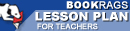Lesson Plans

# Prisoner's Dilemma Test | Mid-Book Test - Easy

This set of Lesson Plans consists of approximately 111 pages of tests, essay questions, lessons, and other teaching materials.
 View a FREE sampleName: _________________________ Period: ___________________

This test consists of 15 multiple choice questions and 5 short answer questions.

## Multiple Choice Questions

1. In what century was Kriegspiel developed?
(a) 19th.
(b) 18th.
(c) 17th.
(d) 20th.

2. In what year was von Neumann invited to join the Manhattan Project?
(a) 1912.
(b) 1954.
(c) 1943.
(d) 1946.

3. How old was von Neumann's daughter from his first marriage when she moved in with him?
(a) Teens.
(c) Pre teens.
(d) Late thirties.

4. Besides Bertrand Russell, what mathematician thought a new war between the U.S. and Soviet Union was inevitable once the conflict ended between the Allied and Axis powers?
(a) John von Neumann.
(b) Merrrill Flood.
(c) Kurt Godel.
(d) John Nash.

5. John von Neumann was from a family of ___ Jews.
(a) Hungarian.
(b) German.
(c) Polish.
(d) Czech.

6. In Chapter 3 we are presented with examples of games that use mixed strategies, but this does not include which game below?
(a) Poker.
(b) Baseball.
(c) Divide the Cake.
(d) Kriegspiel.

7. When the equilibrium solution is used in the prisoner's dilemma game model it leads to mutual ____.
(a) Defection.
(b) Cooperation.
(c) Benefits.
(d) Punishment.

8. John von Neumann's original game theory was extended to find an equilibrium solution to what kind of games?
(a) Non-zero sum, two person games.
(b) Two-person, zero-sum, and non-zero sum games.
(c) Zero-sum, two-person games.
(d) N-person, zero-sum, and non-zero sum games.

9. In Chapter 1, as time runs out for the loved ones, each in a separate room what will each be tempted to do?
(a) Wait it out.
(b) Beg for more time.
(c) Quit.
(d) Push the button.

10. RAND operations were located where?
(a) Virginia.
(b) D.C.
(c) California.
(d) Illinois.

11. What is the name of von Neumann's daughter from his first wife?
(a) Marianne.
(c) Mariana.
(d) Martha.

12. In the divide the cake game the chooser seeks to minimize the ___ piece of cake the cutter will receive.
(a) Minimum.
(b) Maximum.
(c) Size.
(d) Amount.

13. The RAND institute at one time had every great U.S. and free world mathematician, physicist and ____ working there.
(a) Geologist.
(b) Philosopher.
(c) Topologist.
(d) Economist.

14. Whose last work was an attempt to design a human brain based computer.
(a) Kurt Godel.
(b) John von Neumann.
(c) John Nash.
(d) David Hilbert.

15. Game theory involves competitive games between perfectly ____ and opposed players.
(a) Rational.
(b) Matched.
(c) Irrational.
(d) Equal.

1. David Hilbert was a great ____.

2. What country did some feel stood in the way of a world government and should be bombed?

3. Russell was the first to suggest a different game model for ___ than prisoner's dilemma.

4. What course topic was von Neumann invited to teach at Princeton?

5. In what country did von Neumann earn his PhD in mathematics?

 This section contains 419 words (approx. 2 pages at 300 words per page) View a FREE sample